[BACK]
 Computers, Materials & ContinuaDOI:10.32604/cmc.2022.031485Article

A Novel Optimized Language-Independent Text Summarization Technique

1Department of Computer Sciences, College of Computer and Information Sciences, Princess Nourah Bint Abdulrahman University, P.O. Box 84428, Riyadh, 11671, Saudi Arabia
2Department of Information Systems, College of Computer and Information Sciences, King Saud University, Riyadh, Saudi Arabia
*Corresponding Author: Hanan A. Hosni Mahmoud. Email: hahosni@pnu.edu.sa
Received: 19 April 2022; Accepted: 30 May 2022

Abstract: A substantial amount of textual data is present electronically in several languages. These texts directed the gear to information redundancy. It is essential to remove this redundancy and decrease the reading time of these data. Therefore, we need a computerized text summarization technique to extract relevant information from group of text documents with correlated subjects. This paper proposes a language-independent extractive summarization technique. The proposed technique presents a clustering-based optimization technique. The clustering technique determines the main subjects of the text, while the proposed optimization technique minimizes redundancy, and maximizes significance. Experiments are devised and evaluated using BillSum dataset for the English language, MLSUM for German and Russian and Mawdoo3 for the Arabic language. The experiments are evaluated using ROUGE metrics. The results showed the effectiveness of the proposed technique compared to other language-dependent and language-independent summarization techniques. Our technique achieved better ROUGE metrics for all the utilized datasets. The technique accomplished an F-measure of 41.9% for Rouge-1, 18.7% for Rouge-2, 39.4% for Rouge-3, and 16.8% for Rouge-4 on average for all the dataset using all three objectives. Our system also exhibited an improvement of 26.6%, 35.5%, 34.65%, and 31.54% w.r.t. The recent model contributed in the summarization of BillSum in terms of ROUGE metric evaluation. Our model’s performance is higher than the compared models, especially in the metric results of ROUGE_2 which is bi-gram matching.

Keywords: Text summarization: language-independent summarization; ROUGE

1  Introduction

The substantial amount of electronic data, in different languages, has increased the difficulty of mining useful information from it. It is difficult for people to read such huge articles information. Thus, it is essential to have a computerized summarization technique to deduce the important and prominent information rapidly. Computerized summarization techniques have been utilized for different fields such as web pages and online forms. For instance, the authors in  suggested a text token extraction to improve search results. The authors in , proposed a text token extracting approach for media analysis.

Language-independent summary extractors are language analysis applications. They target the generation of shorter text from single or multi-text documents while maintaining the meaning. Summarization techniques can be categorized according to the input, language, approach or the output as depicted in Fig. 1 [3,4]. The summarization can be performed on the input of a single text documents or multi text documents. A set of correlated text documents is utilized in the multi-text documents summarization. A one-text documents source will not show inconsistencies, however, in multi-text documents source conflict and redundancy can be found. Therefore, multi-text documents source summarization is more difficult than single source text documents . Also, the summarization output can be nonspecific that discourses a huge community, or can be text token-based which emphasizes on specific subjects associated with the text token. This can be significant in categorizing the technique into indicative process [3,4].Figure 1: Summarization techniques parameters (input, language, output)

The summarization process can also be defined as extractive where the summarization output is made by choosing the main phrases based on linguistic features and statistical aspects to produce the Weighted-Sum based solutions . While, abstracts depend on analyzing the text semantics using natural language processing techniques to produce new phrases that grasps the main ideas in the source text documents [3,4]. The abstracts synopses are more comprehensible and similar to summaries done by humans, but they need profound knowledge of the source text and also need parsers and text generators [6,7]. Deep learning and transfer learning can be utilized in abstract summarization. Deep learning can usually yield good results. Extract-summarization chooses the important phrases utilizing predefined features. The selected phrases are then combined to produce the summarization output. In multi-text documents, the redundancy problem is raised because phrases are mined from several text documents. Redundancy has to be handled in such cases. Also, restricted summarization needs to choose the best chosen summarization output and not the preeminent phrases. Therefore, multi text documents summarization will lead to a global optimization requirement .

The contributions of this paper are summarized as follows:

1.    This paper proposes a language-independent extractive summarization technique.

2.    The proposed technique presents a clustering-based optimization technique.

3.    The clustering technique determines the main subjects of the text, while the proposed optimization technique minimizes redundancy, and maximizes significance.

4.    Experiments are devised and evaluated for different languages to prove the independent feature of the model

5.    The experiments are performed on datasets in the languages English, German, Russian and the Arabic languages.

This paper comprises the following: surveys of multi-text documents summarization techniques is presented in Section 2. Section 3 introduces problem definition and mathematical representation. Section 4 proposes the new methodology. In Section 5, results are demonstrated. Section 6 depicts conclusion and future work.

2  Related Work

Language-independent text summarization is categorized into two main methodologies: classical and deep learning techniques .

2.1 Classical Techniques

Classical extractive techniques are classified into two techniques. The first approach is the greedy algorithm which chooses a single phrase at a time. The second one is the global technique which examines the best summarization output in place of the best chosen phrases. Such optimization algorithms are NP-hard, and they need heuristics algorithms such as population . Various algorithms are presented for fast summarization techniques.

In Greedy technique, a single phrase is selected using a pre-selected features to be incorporated in the summary. This technique is time-efficient and unpretentious, but has the limitation of not yielding an acceptable summarization because of data redundancy. Many algorithms are presented in this technique such deep learning techniques.

Statistical algorithms are usually utilized in language-independent text summarization using relevance score and statistical classifier . In this techniques, they utilized features such as Term Frequency, phrase position and length to mirror the significance of a phrase in the source file . Theses algorithms are utilized for multiple source text documents summarization. They are utilized also to improve the mixture of the important phrases and to exclude redundancy. The main problem is the lack of understanding the semantic of the text .

On the other hand, in machine learning methods, language-independent models are producing bi-arrangement output. Multiple texts and their extracts are utilized in the learning-phase data. Single phrase is categorized as a summarization output phrase using statistical and/or semantic set of features . According to the authors in , machine learning techniques are well fit for the summarization of text documents. Studies have presented the efficiency of this technique , but, it need labeled data for supervised training, which is time-consuming.

Global summarization explores the best summarization output and not the phrases. This technique yields better output than stochastic technique, but it requires more computational complexity. Many algorithms are employed in this technique such as clustering approaches. The problem, with this approach, is the lengthy time required to obtain the summary.

In Graph, approaches, each text document is stored as a tree with a set of nodes, and set of arcs between the vertices. Each single phrase is represented as vertex, and each edge represents the connection between two related phrases. Each edge is assigned a weight that resembles the two vertices similarity. They utilize dice coefficient metric to represent the resemblance degree of any two phrases. An edge is defined when the resemblance degree is larger than the threshold . This approach is used for text token summarization and requests that phrases are only selected from relevant sub-graph . The main problem with graph techniques is the lack to understand the source text as it utilizes statistical metrics.

2.2 Deep Learning Techniques

Deep learning and neural text summarization achieve better results than classical methods . Deep learning techniques involve a lengthy training phase. Extractive and abstractive deep learning techniques trail the same pipeline as follows:

I.   Words are represented as continuous vector using Word2vec-alike algorithms.

II.  Text documents are encoded utilizing word embedding techniques which encode and extract text features

III. The text representation is added as an input to regression simulations to rank phrases (extractive) or decoders to generate phrases (abstractive) .

Abstractive models focalize on salient features caption (meaning) and then create an abstract like human-written abstracts. Phrase-to-phrase recurrent neural network (RNN) architectures are the prevailing framework for abstract summarization . In this model, an encoder symbolizes token in the input, the decoder generates the summary of the vector encoded. A beam search process is utilized to capture the best sequence to generate the summary .

As a summary, the main challenges for the Arabic summarization process, are the lack of multi-phrase sets for abstract summarization and the difficulty of the Arabic language. Another problem is that ROUGE metric is not a measure of relevance. The ROUGE metric uses exact word matching, while abstracts may rephrase some words into different ones with similar meanings. Also, abstracts may create fake facts, as 35% of abstracts summaries created by this technique face this problem. Other challenges such as phrase repetition and inaccuracy are also stated .

3  Problem Definition

The phases of the proposed system are as follows:

a)   The first phase is the pre-processing phase which comprises tokenization, stop-tokens deletion of the related text documents sources to convert the source text into a unified one.

b)   The second phase defines the informative features and extracts the new representation from each single phrase as a depiction of the significance score.

c)   The third phase uses the C-Means clustering technique to classify the key themes in the source texts.

d)   The next phase utilizes a multi-objectives optimization algorithm to maximize coverage and significance.

e)   We evaluated the presented technique using BillSum , MLSUM , and Mawdoo3  datasets, and the results revealed that our technique performs better than other peer systems.

In the following subsections, we are going to discuss the problem definition and representation of multiple text documents text summarization.

3.1 Problem Formulation

Text documents D=(D1,D2,Dm), where, m is the related text files count. A text document has a group of phrases P(Di)=(P1,P2,Pn), where n is the count of phrases in Di. The objective is to generate a text summary DD where, D represents selected phrases from the set D.

The summarization objectives are defined as follows:

a)   Relevance is defined as the choice of high score relevant and informative phrases from the set D.

b)   Coverage is defined as the choice of phrases that cover important subtopics from the set D to include all the original information.

c)   Redundancy ensures that the selected phrases do not contain redundant repeated information.

d)   Length of the created summary, compared to the original text documents, should have enough length ratio, which should be defined in progress to optimize coverage and redundancy.

3.2 Phrase Mathematical Representation

Phrase mathematical representation is the key task of the language processing procedures. It encodes phrases into vectors. Numerous algorithms were defined for phrase mathematical representations, such as word embedding . In related text documents, each single phrase is defined by a real-valued bag-of-words. Let T=(T1,T2,Tm) defines all unique words in a text documents D, m is the count of non-similar words. Each signal phrase Pj=(Wj1,Wj2,Wjm) is defined as a m-dimensional vector, Wjk is defined as the weight of word tk in phrase Pj. The term’s weight is computed utilizing inverse term frequency process (ITF) . ITF is a distinct version of a process that is used to define significant content in a text documents in the corpus. ITF is normalized by dividing its value to the total text documents count enclosing the term k. ITF process syndicates term frequency with the inverse phrase frequency value, to compute the compound weight for each single term in each single phrase. ITF is utilized to identify the local importance of the word in each phrase. The ITF of tk in phrase Pj is computed as follows:

ITFj=ITFkj×log2(Nnk)(1)

where, ITFj indicates the occurrences count of the term tk in the single phrase Pj. The symbol N denotes the total phrases’ count, and nk is the total number of phrases including the term tk. ITF metric performance is less than word embedding technique. However, word embedding techniques need huge structured data set and are essentially utilized in abstract summarization deep learning methods. Our proposed approach is an extractive technique which doesn’t understand the phrase semantics.

4  The Proposed Language-Independent Text Summarization Technique

In this section, we are proposing a multi-documents Language-independent extractive summarization technique that engages a multi-objective optimization and clustering techniques. The proposed model extracts the most substantial phrases that represent the key topics of the original text while eradicating redundancy of the created text. Fig. 2 displays important phases of the model. The text contents are converted to a combined text document. In the second phase, phrases will be analyzed as bag vectors with the ITF algorithm. Then, a group of features will be selected to prompt the best score of each phrase. Clusters will be formed to detect the subjects that reside in the documents. In the last phase, the relevant summary is computed by an optimization technique that maximizes significance, coverage and also diversity.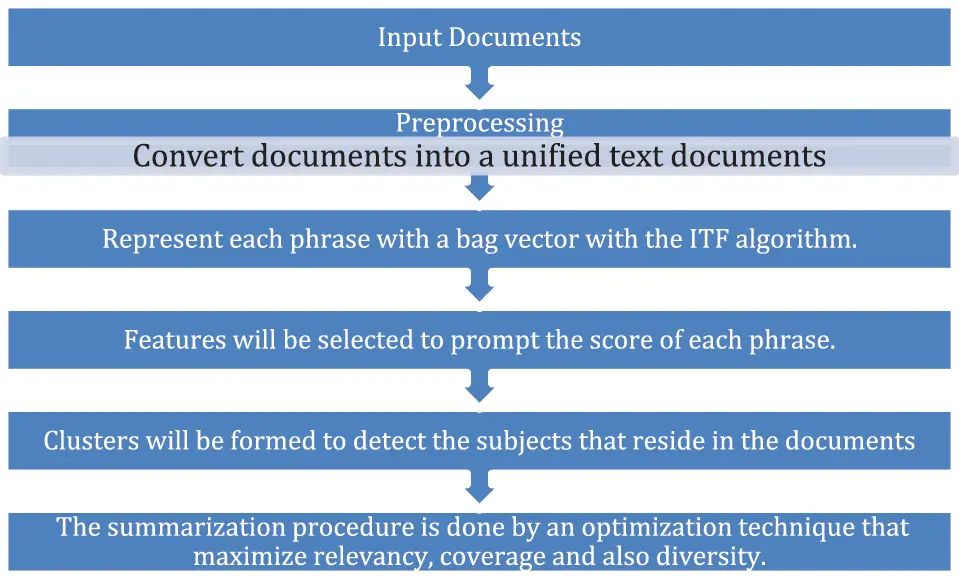Figure 2: Flow diagram of the proposed model phases. Phrases are analyzed as bag vectors and a group of features are selected to compute the best score of each phrase

4.1 Text Preprocessing Phase

The Preprocessing phase targets different language processing challenges by converting the source text documents to simplify the using of the other phases as calculating phrase like hood value. Preprocessing also targets the elimination of the words ambiguity and inconsistency for a better representation. This phase contains two processes namely: tokenization and normalization followed by stop-token removal . We depend on recent studies to select the best preprocessing procedures. We also select CoreNLP language processing tools to aid in these preprocessing methods. We investigated experimentally the outcome of various tokenization processes on text summarization, without dealing with like typos and mistakes issues.

4.1.1 Tokenization

Tokenization targets the splitting of the text documents into smaller partitions such as phrases, and words and sometimes paragraphs . This process is related to morphological study process. This process is usually difficult when handling rich languages that have complicated morphology such as Arabic language. Text tokenization is executed at the phrase and word levels to calculate the phrase score, which utilize the punctuation“.,!,;, and ?”. Tokenization is used to denote phrases as a set using space. We also investigate the semantic tokenization process using CoreNLP of punctuation marks delimiters. This approach is beneficial in regards of the presence of punctuation errors. Tab. 1 displays an input text with phrase tokenization via punctuation and semantic processes. Punctuation tokenization yields two phrases while semantic tokenization outputs one phrase.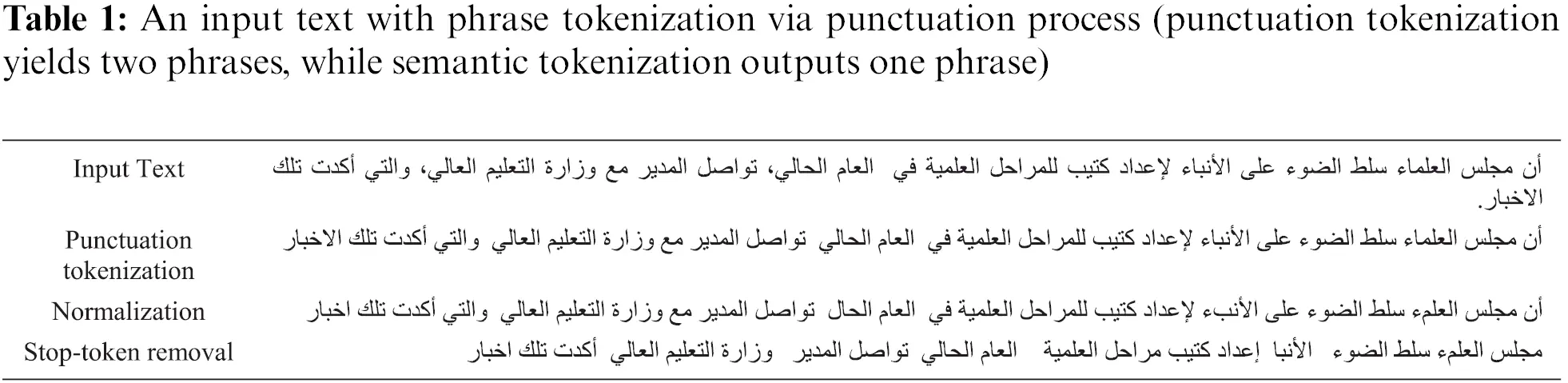4.1.2 Phrase Length

Phrase length can compute the information contents in a phrase. Lengthy phrase is a metric of high information content. It totals the count of words in a phrase divided by the size of the lengthiest phrase, which is computed mathematically as:

length(Pi)=No.oftermsinPiNo.ofwordsinthelongestphrase(2)

Numerous methods are proposed to figure the phrase score , the performance relates phrase-based voting techniques such as BordaFuse and CombANZ. We approve, for all the features, a linear sum of normalized scores to estimate each phrase value as follows:

Score(pi)=h1titlesimilarity(pi,t)+h2keywords(pi)+h3location(pi)+h4length(pi)(3)

The weight hi of a feature replicates its rank and therefore impact the calculation of the total rank. using results, we define ranks as follows: h1 = 3, h2 = 2, h3=1, and h4=12.5 .

4.2 Topics Selection for Clustering

Related text documents usually have the same group of subjects. To define these subjects, we will have employed clustering algorithm . We selected a partitioning algorithm (C-Means). This method is extensively used to defeat the shortcomings of the K-means algorithm. C-Means employs nonlinear similarity measures for cluster’s representation. (Ci) represents a point in the cluster with a minimum dissimilarity with other points (Oi) in the cluster. (Ci) is a point that is analogous in concept to centroids, but still is a point in the data set. The dissimilarity (Ci, Oi)  is computed by utilizing any distance metric like Rectilinear distance d (Abs (Ci(Oi)). The C-Means algorithm selects the cluster centroid that reduces the sum of the d from this centroid. If k is the count of clusters, P is the group of phrases in the texts represented using its ITF algorithm. Cj is the centroid of the cluster kj. The C-Means process for phrase clustering is depicted by the following :

A.  Define K predefined clusters.

B.  Select k-phrases, randomly, from P to be the initial centroids.

C.  Assign each phrase Pi to the predefined cluster Kj with the most adjacent centroid using Rectilinear distance, PiKj,  where |CiPi| is the minimum value for all Kj.

D.  Re-compute the centroid Cj for the predefined Kj by selecting the phrases that reduce the sum w.r.t all other phrases, select Cj where:

Sum of differences=Cj siCj, where |Cisi|is minimum

E.  Repeat steps A and B until the value of the centroids become constant.

The count of predefined k defines the count of various subjects that are present in the text files. Since k is predefined by the consumer, it can be time inefficient to acquire its minimum value. Therefore, we used the elbow algorithm to determine the optimal value of k. Elbow measure defines cluster cohesion, and how close are the enclosed objects. On the other hand, separation defines the mutual exclusion metric between clusters. For each phrase Pi, a(i) is defined as the mean distance between Pi and all other phrases in Kj. CCi, define d(si, C) as the mean distance between Pi, and the objects O in cluster Kj. Then compute d(si, C)  CCi. b(i) is the least distance. The elbow_measure of a phrase Pi is calculated as follows:

elbow_measure(Pi)=b(i)a(i)max(b(i),a(i))(4)

a) Defining the objective functions

We define multi objective functions that are used to rank the generated solutions. They are coverage and significance. Also, we include diversity objective to produce better summarization. The objectives are defined as follows:

•   Coverage ensures that the summary includes all essential contents and important subjects that occur in the texts. Coverage is depicted as the similarity between phrases Pi where Pi is an element in both the Summary and the documents’ topics. This similarity is defined by the centroids C of the generated clusters. Therefore, the function fcoverage is maximized as follows:

fcoverage(X)=PiSummaryCjdice (Pi,Cj)(5)

where, dice  is defined as the dice coefficient metric, Pi represent the ith phrase, Cj is the centroid of the cluster Kj.

•   Diversity goal is to decrease redundancy in the output summary by not repeating the same information using multiple phrases. We compute the redundancy using the similarity measure between phrases in the generated summary:

fredundancy(X)=i=1N1j=1N1dice (Pi,Pj)(6)

where, dice is the dice coefficient metric and N1 is the count of phrases in the generated summary. The model attempts to minimize redundancy to produce a better summary.

Crossover is utilized to enhance diversity in the population . Crossover models have been proposed, such as the binary crossover which utilizes probability density to simulate the crossover operator of the phrase representation .

•   Significance is calculated by the phrase score and describes the importance phrases in the generated summary. We intend to maximize the significance as an objective, that support the phrases with higher rank to be enclosed. We compute the significance objective as follows:

fsignificance(X)=PiSummaryScore(Pi)(7)

Finally, we can say that the proposed model is an optimization problem defined as following:

fmodel(x)=max(fcoverage,fsignificance,1fredundancy )(8)

b) Generate text summarization

The optimization procedure produces a number of maximum Weighted-Sum solutions. Thus, we have to choose the best solution utilizing user requirements. We use a majority voting algorithm to aggregate the solutions . To produce the final solution, we implement the majority voting algorithm over every dominated solution. The summary is generated by selecting the phrases that gain majority as solutions as depicted in Tab. 2. When the majority output is lengthy, we will delete phrases with low scores. The process is depicted in Tab. 3.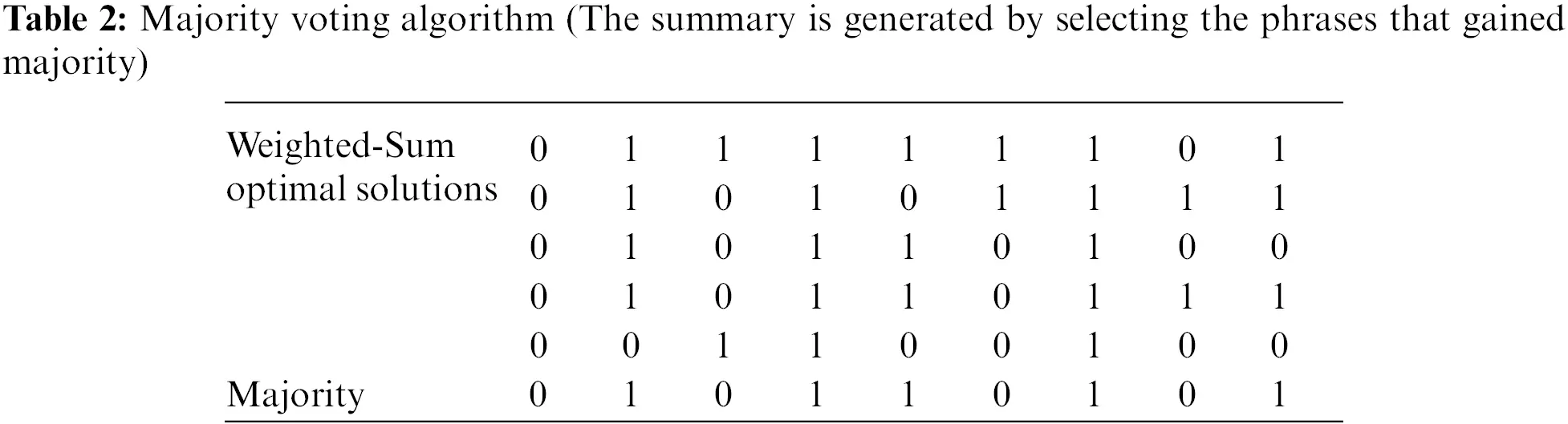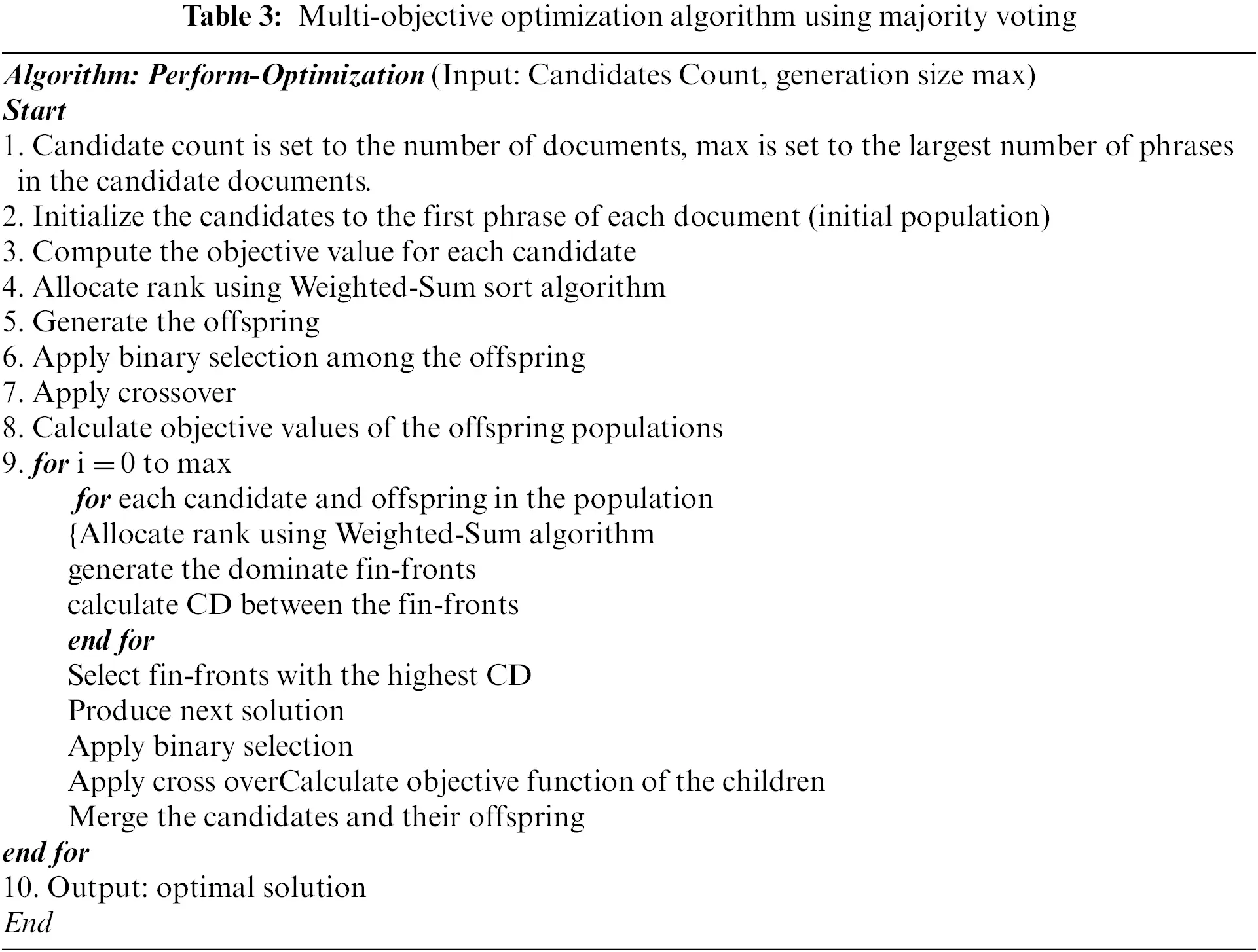5  Experiments

In this section, we are evaluating the efficacy of our summarization model by describing the conducted experiments. Datasets are described in Section 5.1. Evaluation metrics are discussed in Section 5.2. The results are reported and discussed in Section 5.3. Finally, we compared our model with other related models in Section 5.4.

5.1 The Dataset

To evaluate our model, we utilized the public datasets BillSum , MLSUM , and Mawdoo3 . These datasets are summarized for English, Arabic, German and Russian languages. Tab. 4 presents the datasets statistics. Where, reference sets are set of reference documents summarized by experts and used as ground truth.5.2 Evaluation Metrics

We utilize the ROUGE evaluation metric  for performance evaluation of our proposed model. ROUGE is an acknowledged metric and is the official metric for computerized evaluation of text-document summarization. ROUGE measures the superiority of the output by calculating similarity score of the output vs. the ground truth summarized by a human. Similarity is calculated by including all overlapping terms using Ngram, term sequences (ROUGE_L) and term pairs (ROUG_SU). In our performance evaluation, we utilize the metrics: ROUGE_N (N= 1, 2, 3 and 4). The ROUGE_N metric matches Ngram of the generated text summary and reference summary and then calculates the count of matches. It is computed as follows:

ROUGE_N=Ssummary(R)NgramSCount(matchedNGram)Ssummary(R)NgramSCount(NGram)(9)

5.3 Experimental Results

The first experiment reveals the performance of the pre-processing techniques in text document summarization. The datasets are tokenized punctually via CoreNLP tool. Tabs. 5 and 6 present the outcome of the pre-processing on the summarization process. They display the results of tokenization alone and tokenization using punctuation. It is obvious from Tab. 5, that the best performance was achieved when the punctuation is utilized in the tokenizer. For the English language, the technique accomplished F-measure of 0.474, 0.304, 0.208, and 0.172, on the average, for ROUGE_1, ROUGE_2, ROUGE_3, and ROUGE_4, respectively. The F-measure results for the other languages are detailed in Tab. 5. In detail, the punctuation tokenizer is very stable given that the data is correctly punctuated, while the tokenizer alone is better for data without punctuations. The used datasets are fully punctuated with full marks. They describe the reason that punctuation tokenizer has better performance than the alone tokenizer. Since the optimization procedure utilizes random variables to control the crossover operators, we will not depend on single run observation. We will compute the mean F-measure of 20-Independent runs. Also, we will employ the same concepts in comparison with similar systems using the same dataset. MLSUM for German and Russian and Mawdoo3 for the Arabic language.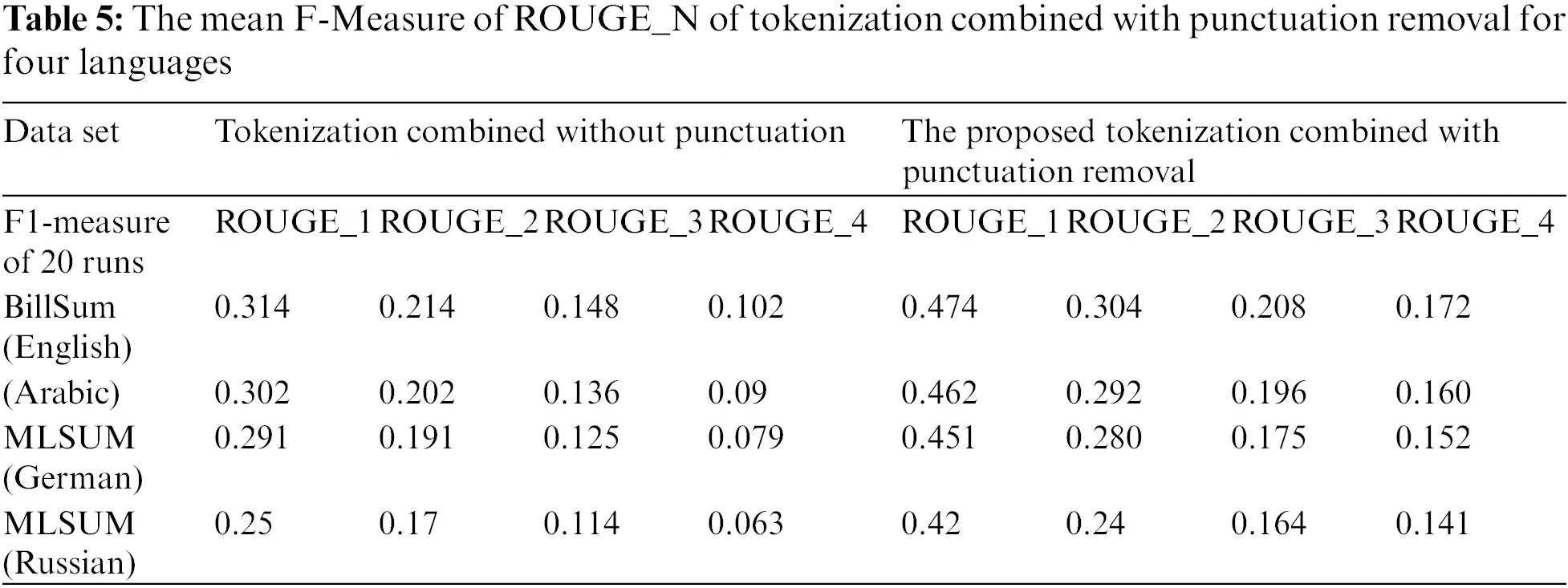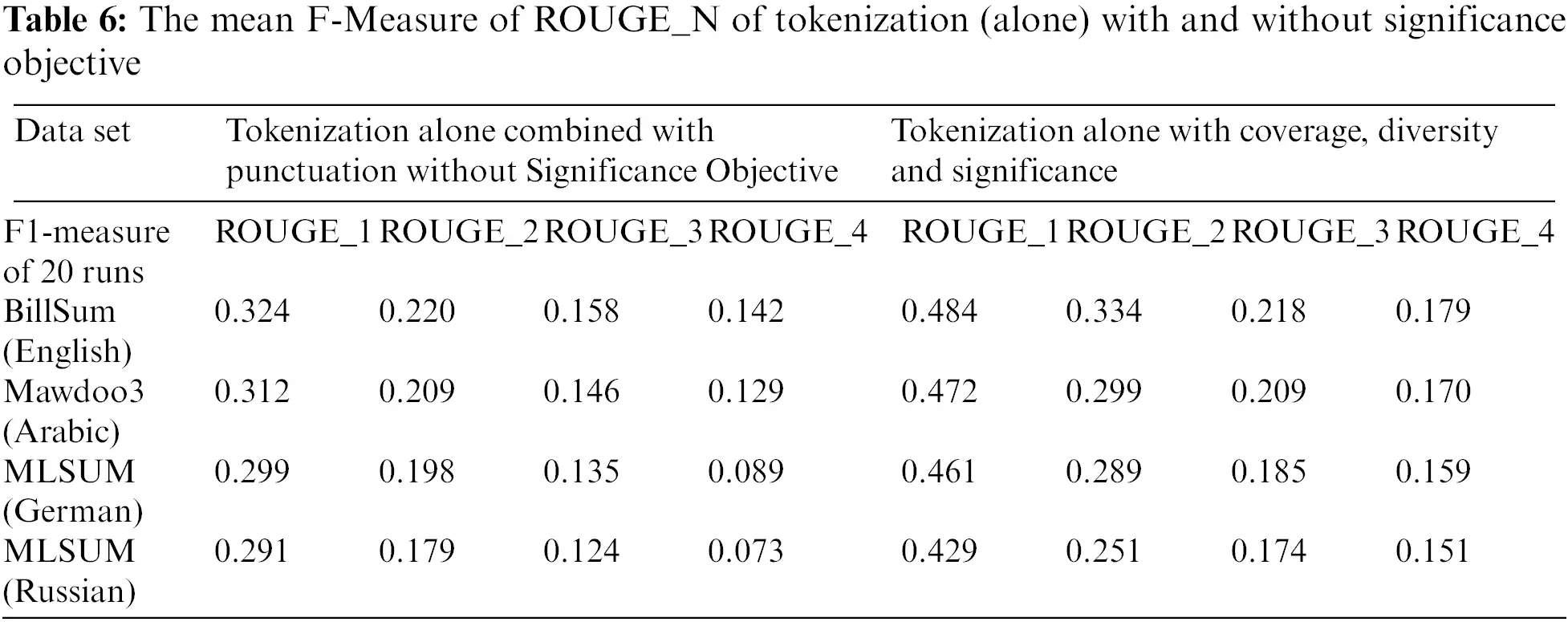The second experiment reveals the performance of maximizing the objective named significance score. Tab. 6 demonstrates the results of maximizing coverage function and diversity function only. Tab. 7 demonstrates the results of maximizing both coverage and diversity, as well as significance. The results, for the English language, prove that maximization increases the efficiency of the results considerably with a mean improvement of 38.5%, 34.8%, 23.4% and 18.1% respectively, as depicted in Tabs. 6 and 7. This increased efficiency stems from the significant features that are included in the significance function such as phrase position, which are not included in either the coverage function or the diversity function.5.4 Comparing with Other Related Works

We conducted an objective study comparing our proposed model against similar language-independent extractive document text summarization published models. The comparison is depicted in Tab. 8 which delivers a comparison of performance of our proposed model against other similar models on BillSum dataset utilizing the ROUGE metric. The results indicate that our model outperforms all other models using BillSum dataset. Our system exhibits an improvement of 26.6%, 35.5%, 34.65%, and 31.54% w.r.t. to the compared models using BillSum in terms of Rouge-N from 1 to 4, respectively. It is found that the proposed model’s accuracy is higher than compared models, from the result of the ROUGE_2 which is bi-gram matching.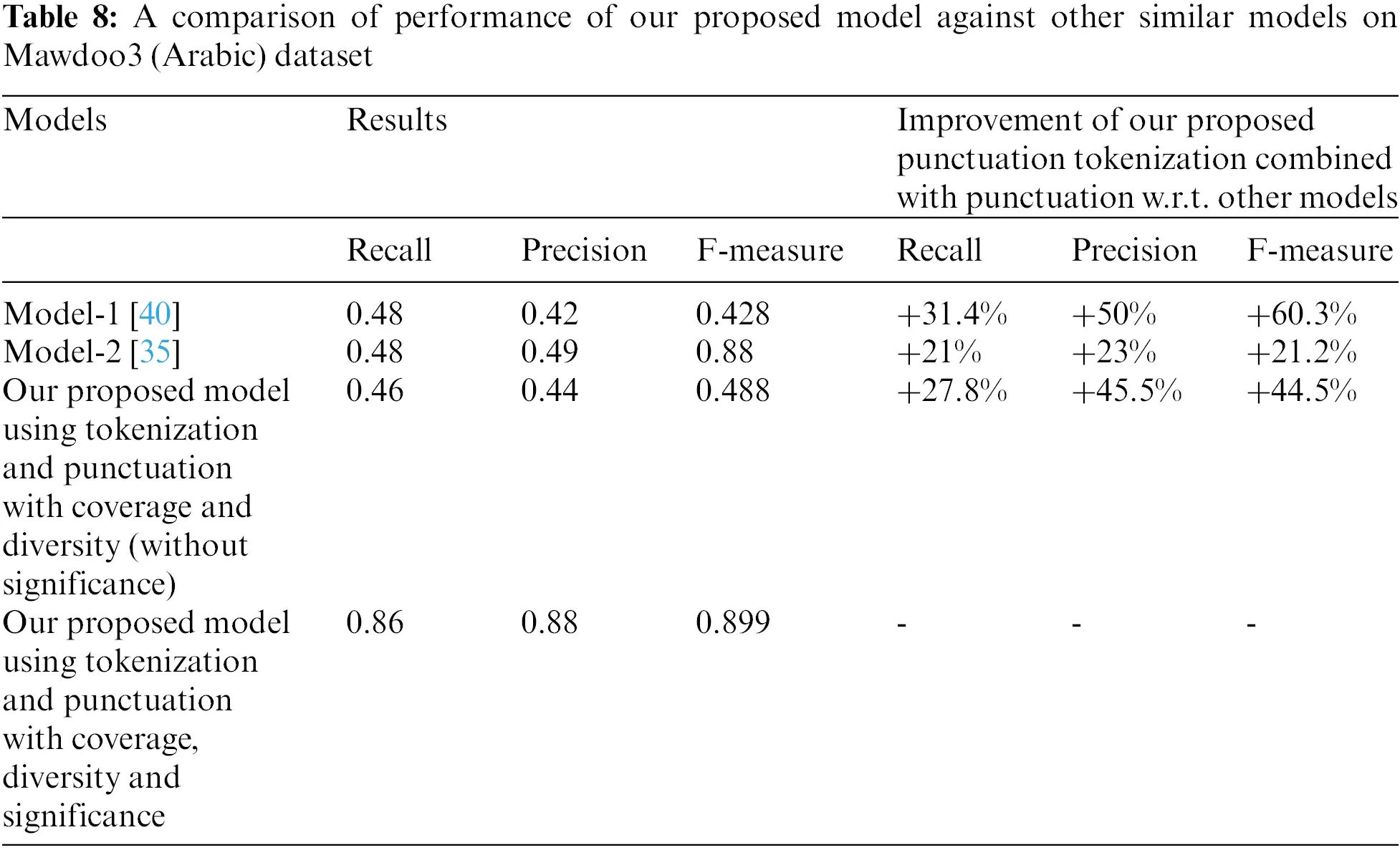5.5 Computational Time Comparison

In this section, we are establishing the computational time required to construct summaries comparing our proposed model (using different parameters including tokenization and punctuation with coverage, diversity and significance) against state of the art models Model-1  and Model-2 . From the database Mawdoo3 (Arabic) (see Fig. 3). As depicted from the figure our model has an advantage in computational time of extracting a phrase in the final summary in seconds vs. Number of phrases in thousands in the Mawdoo3 (Arabic) Reference sets.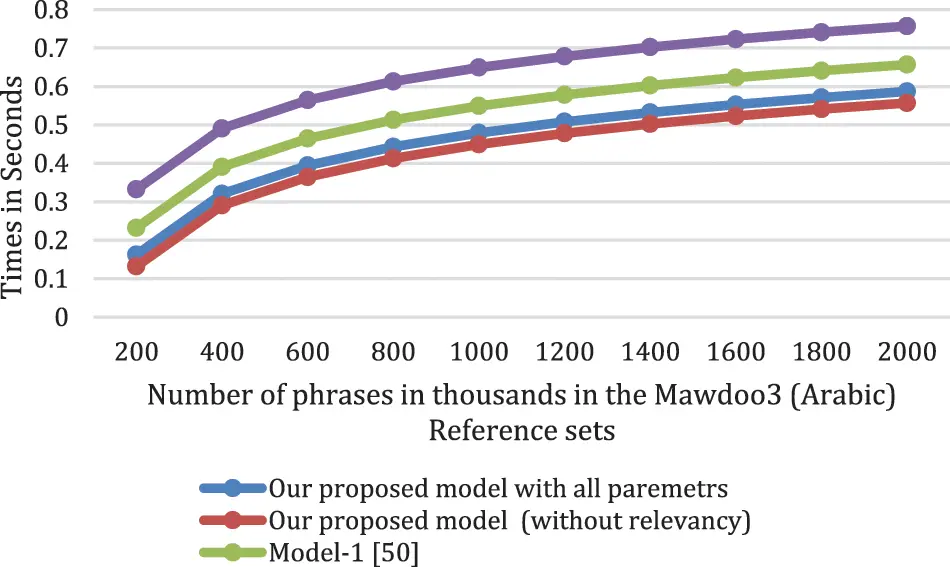Figure 3: Average time to extract a phrase in the final summary in seconds vs. Number of phrases in thousands in the Mawdoo3 (Arabic) Reference sets

Experiments establish that the cost of extracting N phrases in the final summary has an upper bound of O (N Log (N)). Therefore, training time is O M (N Log (N)). We computed the CPU time that our model consumed to form the final summarization text, as depicted in Fig. 4.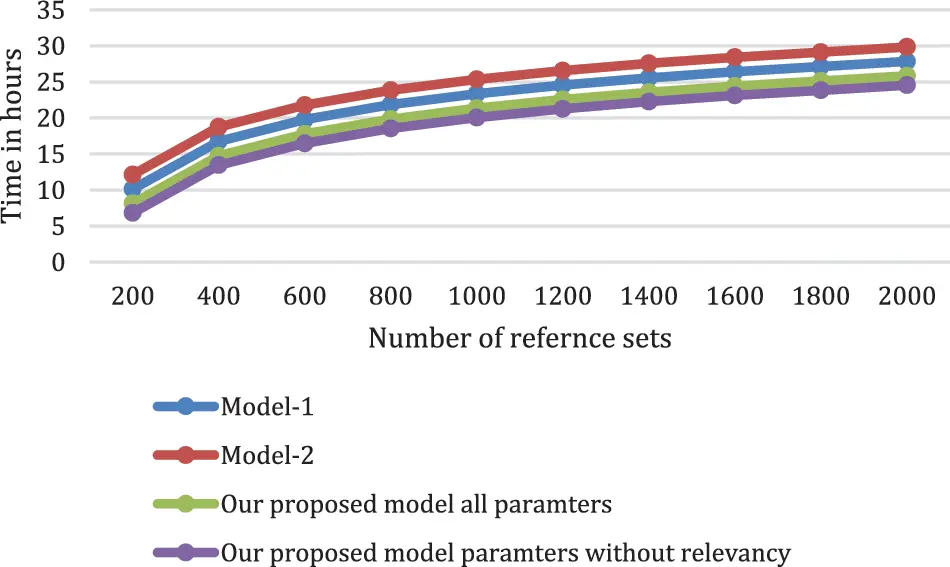Figure 4: The comparison of the cost of average CPU time for training phase for different models in hours

5.6 Discussion

The experiments depicted the performance of maximizing the significance score, coverage function and diversity function. The results demonstrated that maximizing both coverage and diversity and increased the efficiency of the results with an average accuracy of 90%. The comparative study of our proposed model against state of the art summarization models using BillSum dataset is depicted using the ROUGE metric. The results indicated that our model outperform all other models with an increase of 35.5 using the ROUGE_2 metric, which is bi-gram matching.

Also, the CPU time of our model has an advantage in extract acting time of a phrase in the final summary vs. other compared models.

6  Conclusion

In conclusion, we formulated the multi-language-independent text summarization process as an objective optimization process (maximize multiple objectives simultaneously). The proposed model employs four phases: the first phase is the preprocessing process followed by feature extraction and clustering, while the last phase is the multi-objectives simultaneous optimization. Sentences are modeled in a unified form through preprocessing such as tokenization, stop word removal and normalization. Statistical features are selected and used for significance scoring for each phrase. Topics of the related documents are defined using centroid clustering. The last phase generates an optimal summary using a multi-objectives optimization evolutionary method, maximizing significance and minimizing redundancy. Results verify the efficacy of our model over the state-of-art models by measuring the ROUGE metric.

Still we have some limitations as follows (i) Sentence score is computed experimentally, it could be computed through Genetic Algorithm, and (ii) We did not include coherency of the output, we could have included it to the objectives to be optimized.

Acknowledgement: We would like to thank Princess Nourah bint Abdulrahman University Researchers Supporting Project number (PNURSP2022R113), Princess Nourah bint Abdulrahman University, Riyadh, Saudi Arabia.

Funding Statement: This research was funded by Princess Nourah bint Abdulrahman University Researchers Supporting Project number (PNURSP2022R113), Princess Nourah bint Abdulrahman University, Riyadh, Saudi Arabia.

Conflicts of Interest: The authors declare that they have no conflicts of interest to report regarding the present study.

## References

1. R. Qumsiyeh and Y. Ng, “Searching web text documents using a classification approach,” International Journal of Web Information Systems, vol. 12, no. 1, pp. 83–101, 2019.
2. A. Pal and D. Saha, “An approach to automatic language-independent text classification using simplified lesk algorithm and wordnet,” International Journal of Control Theory and Computer Modeling (IJCTCM), vol. 3, no. 4/5, pp. 15–23, 2020.
3. 4.M. Al-Smadi, S. Al-Zboon, Y. Jararweh and P. Juola, “Transfer learning for arabic named entity recognition with deep neural networks,” IEEE Access, vol. 8, pp. 37736–37745, 2020.
4. M. Othman, M. Al-Hagery and Y. Hashemi, “Arabic text processing model: Verbs roots and conjugation automation,” IEEE Access, vol. 8, pp. 103919–103923, 2020.
5. H. Almuzaini and A. Azmi, “Impact of stemming and word embedding on deep learning-based arabic text categorization,” IEEE Access, vol. 8, pp. 27919–27928, 2020.
6. M. Alhawarat and A. Aseeri, “A superior arabic text categorization deep model (SATCDM),” IEEE Access, vol. 9, pp. 24653–24661, 2020.
7. S. Marie-Sainte and N. Alalyani, “Firefly algorithm based feature selection for arabic text classification,” Journal of King Saud University Computer and Information Sciences, vol. 32, no. 3, pp. 320–328, 2020.
8. T. Sadad, A. Rehman, A. Munir and T. Saba, “Text tokens detection and multi-classification using advanced deep learning techniques,” Knowledge-Based Systems, vol. 84, no. 6, pp. 1296–1908, 2021.
9.    M. El-Affendi, K. Alrajhi and A. Hussain, “A novel deep learning-based multilevel parallel attention neural (MPAN) model for multidomain arabic sentiment analysis,” IEEE Access, vol. 9, pp. 7508–7518, 2021.
10. Y. LeCun, Y. Bengio and G. Hinton, “Deep learning,” Nature, vol. 521, no. 7553, pp. 436, 2020.
11. S. He, Z. Li, Y. Tang, Z. Liao, F. Li et al., “Parameters compressing in deep learning,” Computers Materials & Continua, vol. 62, no. 1, pp. 321–336, 2020.
12. W. El-Kassas, C. Salama, A. Rafea and H. Mohamed, “Automatic text classification: A comprehensive survey,” Expert Systems Applications, vol. 5, no. 3, pp. 123–131, 2021.
13. M. Gomez, M. Rodríguez and C. Pérez, “Comparison of automatic methods for reducing the weighted-sum fit-front to a single solution applied to multi-text documents text classification,” Knowledge-Based Systems, vol. 10, no. 1, pp. 173–196, 2019.
14. A. Widyassari, S. Rustad, G. hidik and A. Syukur, “Review of automatic language-independent text classification techniques & methods,” Journal King Saud University of Computer Information Sciences, vol. 19, no. 1, pp. 133–142, 2020.
15.  M. Lins, G. Silva, F. Freitas and G. Cavalcanti, “Assessing phrase scoring techniques for tokenization text classification,” Expert Systems Applications, vol. 40, no. 14, pp. 755–764, 2019.
16.  Y. Meena and D. Gopalani, “Efficient voting-based tokenization automatic text classification using prominent feature set,” IETE Journal of Reasoning, vol. 62, no. 5, pp. 581–590, 2020.
17.  A. Al-Saleh and M. Menai, “Automatic arabic text classification: A survey,” Artificial Intelligence Review, vol. 45, no. 2, pp. 203–234, 2020.
18.  V. Vijayan, K. Bindu and L. Parameswaran, “A comprehensive study of text classification algorithms,” Advances in Informatic, vol. 2, no. 1, pp. 1109–1113, 2019.
19. A. Al-Saleh and M. Menai, “Solving multi-text documents classification as an orienteering problem,” Algorithms, vol. 11, no. 7, pp. 96–107, 20
20. V. Patil, M. Krishnamoorthy, P. Oke and M. Kiruthika, “A statistical approach for text documents classification,” Knowledge-Based Systems, vol. 9, pp. 173–196, 20
21. H. Morita, T. Sakai and M. Okumura, “Query pnowball: A co-occurrence-based approach to multi-text documents classification for question answering,” Information Media Technology, vol. 7, no. 3, pp. 1124–1129, 20
22. D. Patel, S. Shah and H. Chhinkaniwala, “Fuzzy logic based multi text documents classification with improved phrase scoring and redundancy removal technique,” Expert Systems Applications, vol. 194, pp. 187–197, 2019.
23. M. Safaya, A. Abdullatif and D. Yuret, “ADAN-CNN for oﬀensive speech identiﬁcation in social media,” Expert Systems, vol. 4, no. 3, pp. 167–178, 2020.
24. W. Antoun, F. Baly and H. Hajj, “ArabADAN: Transformer-based model for arabic language understanding,” Information Technology, vol. 4, no. 3, pp. 124–132, 2020.
25. M. Fattah and F. Ren, “GA MR FFNN PNN and GMM based models for automatic text classification,” Computer Speech Languages, vol. 23, no. 1, pp. 126–144, 2021.
26. A. Qaroush, I. Farha, W. Ghanem and E. Maali, “An efficient single text documents arabic language-independent text classification using a combination of statistical and semantic features,” Knowledge-Based Systems, vol. 7, no. 2, pp. 173–186, 2019.
27. V. Gupta and G. Lehal, “A survey of language-independent text classification tokenization techniques,” Journal Emerging Technology Web, vol. 2, no. 3, pp. 258–268, 2020.
28. I. Keskes, “Discourse analysis of arabic text documents and application to automatic classification,” Algorithms, vol. 12, no. 3, pp. 187–198, 2019.
29. X. Cai, W. Li and R. Zhang, “Enhancing diversity and coverage of text documents summaries through subspace clustering and clustering-based optimization,” Information Science, vol. 279, pp. 764–775, 2021.
30. BillSum database https://www.tensorflow.org/datasets/catalog/billsum.
31. MLSUM database: https://github.com/huggingface/datasets/tree/master/datasets/mlsum.
32. Arabic database: https://github.com/mawdoo3.com.
33. W. Luo, F. Zhuang, Q. He and Z. Shi, “Exploiting relevance coverage and novelty for query-focused multi-text documents classification,” Knowledge-Based Systems, vol. 46, pp. 33–42, 2019.
34.  A. Zhou, B. Qu, H. Li and Q. Zhang, “Multiobjective evolutionary algorithms: A survey of the state of the art,” Swarm Evolutionary. Computation, vol. 1, no. 1, pp. 32–49, 2020.
35. M. Sanchez, M. Rodríguez and C. Pérez, “Tokenization multi-text documents text classification using a multi-objective artificial bee colony optimization approach,” Knowledge-Based Systems, vol. 159, no. 1, pp. 1–8, 2020.
36. P. S. Lakshmana and S. Kalpana, “UNL based document summarization based on levels of users,” International Journal of Computer Applications, vol. 66, no. 2, pp. 167–177, March 2020.
37. G. Lins, F. Silva, A. Freitas and G. Cavalcanti, “Assessing phrase scoring techniques for extractive text summarization,” Expert System Application, vol. 40, no. 14, pp. 5755–5764, 2013.
38. S. Mangairkarasi and S. Gunasundari, “Semantic based text summarization using universal networking language,” International Journal of Applied Information Systems, vol. 3, no. 1, pp. 34–45, 2019.
39. W. El-Kassas, C. Salama, A. Rafea and H. Mohamed, “Automatic text summarization: A comprehensive survey,” Expert Systems Application, vol. 3, no. 1, pp. 123–132, 2021.
40. M. Sanchez-Gomez, A. Vega-Rodríguez and J. Pérez, “Comparison of automatic methods for reducing the weighted-sum fit-front to a single solution applied to multi-text documents text summarization,” Knowledge Based Systems, vol. 1, no. 2, pp. 123–136, 2019.This work is licensed under a Creative Commons Attribution 4.0 International License, which permits unrestricted use, distribution, and reproduction in any medium, provided the original work is properly cited.